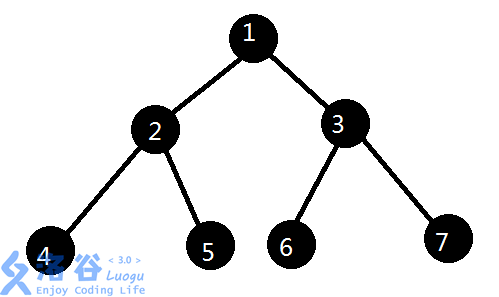柒葉灬 的博客柒葉灬 的博客

线段树合并（个人笔记15）

posted on 2018-12-09 22:09:13 | under 专题总结 |

线段树合并

模板

int merge(int x,int y){
if(!x||!y)return x|y;
lson[x]=merge(lson[x],lson[y]);
rson[x]=merge(rson[x],rson[y]);
sum[x]+=sum[y];
return x;
}

时间复杂度（重点！！！）• $4,5,6,7$ : 第三层每一个点最多被遍历 $1$ 次，总共 $4$ 次
• $2,3$ : 第二层每一个点最多被处理 $2$ 次，总共 $4$ 次
• $1$ : 第一层的这个点最多被处理 $4$ 次，总共 $4$ 次。

END